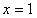Method 1

There are two methods for solving exponential equations. The first method requires that the bases in both exponentials are the same. If the two sides of the original equation do not have the same base, it is sometimes possible to rewrite them with the same base. This method uses an exponential property which states:

If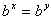then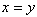.

Example 1 Solve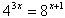Step 1. Rewrite each side with a common base.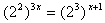Step 2. Use the Power of a Power Property.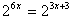Step 3. Since the bases are the same, equate the exponents.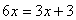Step 4. Solve for x.